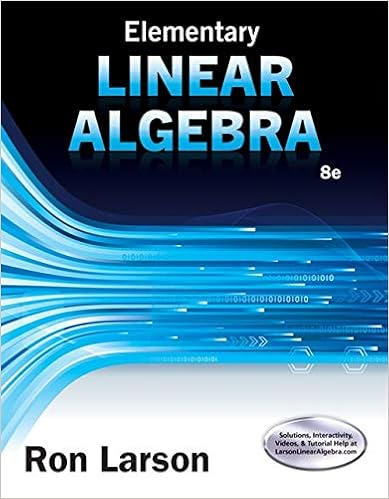# What is the standard equation of a circle at the

• Test Prep
• 46
• 99% (112) 111 out of 112 people found this document helpful

This preview shows page 29 - 32 out of 46 pages.

##### We have textbook solutions for you!
The document you are viewing contains questions related to this textbook.The document you are viewing contains questions related to this textbook.
Chapter 1 / Exercise 15
Elementary Linear Algebra
LarsonExpert Verified
62) What is the standard equation of a circle at the origin?@ x2+ y2= r2a. x2- y2= r2*b. x2+ y2= r2c. x2+ y2= rd. x2- y2= r
63) In the standard equation of a circle (x – h)2+ (y – k)2= r2, the radius is represented by r2and the center of the circle is represented by (-h, -k).
2+ (y – k)2= r2, the radius is represented by r2and the center of the circle is represented by (h, k).
Objective 9: Students will be able to write the standard equation of a circleDisplay 1 Type: E
##### We have textbook solutions for you!
The document you are viewing contains questions related to this textbook.The document you are viewing contains questions related to this textbook.
Chapter 1 / Exercise 15
Elementary Linear Algebra
LarsonExpert Verified
64) Write the standard equation of a circle.
65) In the standard equation of a circle, what represents the center of the circle and the radius?
Objective 10: Students will be able to write the equation of a transformed circleDisplay 2 66) Pizza Planet delivers pizza within a 17 mile radius of their store. If this area is represented graphically, with Pizza Planet located at (2, 4), what is the equation that represents the delivery area?
67) The hospital provides ambulance service within a 60 mile radius of the hospital. If this servicearea is represented graphically, with the hospital located at (0, 0), what is the equation that represents the service area?
68) Write the standard equation of the circle with center (-3, 5) and a diameter of 18.
69) Write the standard equation of the circle with center (-8, -7) and a radius of 6.5.@ (x + 8)2+ (y + 7)2= 42.25a. (x + 8)2+ (y + 7)2= 6.5b. (x – 8)2+ (y – 7)2= 6.5*c. (x + 8)2+ (y + 7)2= 42.25d. (x – 8)2+ (y – 7)2= 42.25
70) The equation of a circle is (x - 3)2+ (y - 5)2= 81. Determine the coordinates of the center of the circle and the length of the radius@ Center (-3, -5), radius is 9
71) The equation of a circle is (x + 4)2+ (y + 2)2= 16. Determine the coordinates of the center of the circle and the length of the radius@ Center (-4, -2), radius is 4
•••